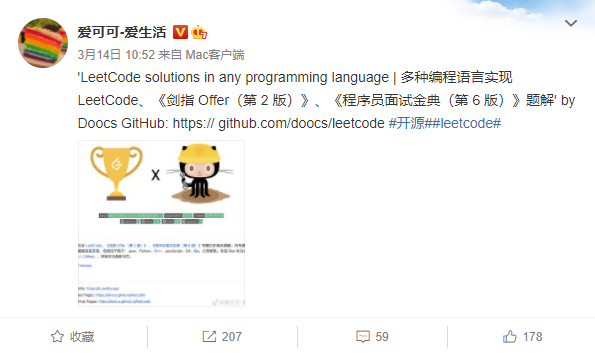LeetCode Offer 2 Offer 6 JavaPythonC++JavaScriptC#Go Star

English Version

### 1.

• - ``
• - ``
• - ``
• - ``
• - ````
• - ``
• - ``
• - - ``
• - - ``
• - ````
• - ````
• - ````
• K - ``
• 1 - ```lowbit`
• - ``

• - ``````
• I - ``
• - ``
• - ``
• - ``
• II - ``
• - ``
• - ``
• - ``
• - ``
• K - ``
• - ````
• II - ````
• - ``
• - ``
• - ````
• - ``

### 3.

• - `BFS`DFS`Flood Fill ```
• - `BFS``Flood Fill`
• 01 - `BFS`
• - `BFS`
• - `BFS```
• - `BFS```
• - `BFS```
• - `BFS```
• - ``` BFS``A* `
• - ``` BFS`
• - ``` BFS`
• - `BFS````A*`
• - `BFS````A*`
• - `BFS``A*`
• - `BFS`
• - `BFS`
• - `DFS````Flood Fill`
• - `DFS`````
• - `DFS`````
• - `DFS`````
• k - `DFS`````
• - `DFS`````
• - `DFS`````

### 4. DP

• - ` DP```
• - ` DP```
• - ` DP```
• II - ` DP```
• - ` DP```
• - ` DP`````
• III - ` DP```
• - ` DP`````
• - `DP`
• - `DP`
• - ` DP```
• ASCII - ` DP```
• - ` DP```
• - `0-1`
• - `0-1`
• II - `0-1`
• - ``
• - ``
• K - ``

• - ````
• - ````
• - ````
• - ``
• - ``
• - ````
• II - ````
• - ````
• - ``
• - - ````
• - ````
• - ````
• K - ``
• - - ````
• - ````
• - ````
• - ``````
• - ````
• - ````
• Range - ````
• III - ````
• - ``````
• - ````
• II - ``````

### 6.

• - ```Dijkstra `Bellman Ford`SPFA `
• - ```Dijkstra `
• - ```Prim ``Kruskal `
• - `Kruskal`
• - `Kruskal`
• - `Kruskal`
• - ````

PR

1. fork GitHub clone
2. leetcode
4. PR review
5. https://help.github.com/cn

Gitpod.io## Stars"You help the developer community practice for interviews, and there is nothing better we could ask for." -- Alan Yessenbayev

@-- 4.0

Get A Weekly Email With Trending Projects For These Topics
No Spam. Unsubscribe easily at any time.
Javascript (1,059,720
Python (807,228
Java (388,471
C Plus Plus (238,799
C Sharp (164,144
Golang (158,264
Github (43,825
Algorithms (37,443
Programming (18,140
Leetcode (3,832
Leetcode Solutions (790